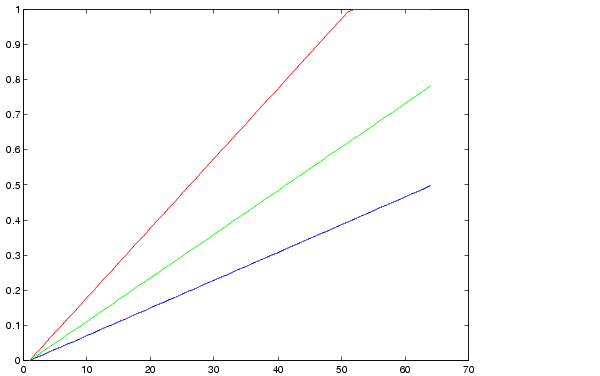MATLAB Function Referencergbplot

Plot colormap

Syntax

• ```rgbplot(cmap)
```

Description

```rgbplot(cmap) ``` plots the three columns of `cmap`, where `cmap` is an m-by-3 colormap matrix. `rgbplot` draws the first column in red, the second in green, and the third in blue.

Examples

Plot the RGB values of the `copper` colormap.

• ````rgbplot(copper)````

• ``````colormaprgb2hsv ribbon